ellipsoid

Create ellipsoid

Description

example

[X,Y,Z] = ellipsoid(xc,yc,zc,xr,yr,zr) returns the x-, y-, and z-coordinates of an ellipsoid without drawing it. The returned ellipsoid has center coordinates at (xc,yc,zc), semiaxis lengths (xr,yr,zr), and consists of 20-by-20 faces.

The function returns the x-, y-, and z- coordinates as three 21-by-21 matrices.

To draw the ellipsoid using the returned coordinates, use the surf or mesh functions.

example

[X,Y,Z] = ellipsoid(xc,yc,zc,xr,yr,zr,n) returns the x-, y-, and z-coordinates of an ellipsoid with n-by-n faces. The function returns the x-, y-, and z-coordinates as three (n+1)-by-(n+1) matrices.

example

ellipsoid(___) plots the ellipsoid without returning the coordinates. Use this syntax with any of the previous input arguments in previous syntaxes.

example

ellipsoid(ax,___) plots into the axes specified by ax instead of the current axes. Specify the axes as the first input argument.

Examples

collapse all

Create and plot an ellipsoid with a center at (0, –0.5, 0) and semiaxis lengths (6, 3.25, 3.25). Use axis equal to use equal data units along each coordinate direction.

ellipsoid(0,-0.5,0,6,3.25,3.25)
axis equal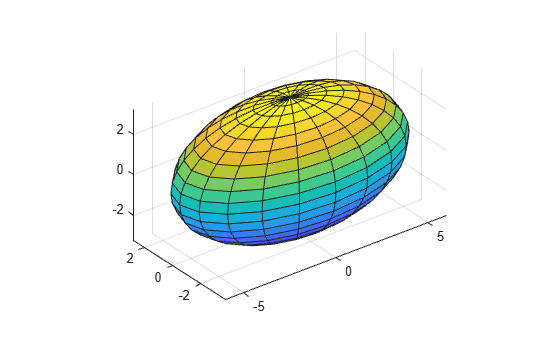Generate coordinates of an ellipsoid with a center at (0, 0, 0) and semiaxis lengths (1.5, 1.5, 3).

[X,Y,Z] = ellipsoid(0,0,0,1.5,1.5,3);

Create a surface plot of the ellipsoid.

surf(X,Y,Z);
axis equal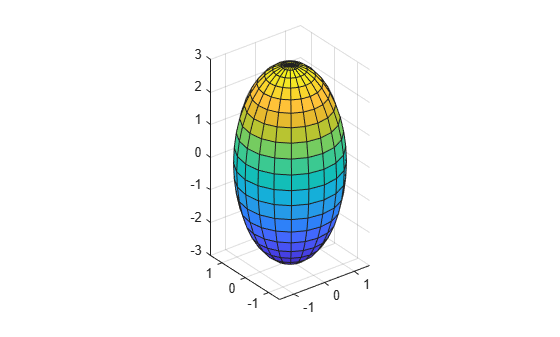Plot a second ellipsoid with its center translated by (3, 0, 5) from the first ellipsoid. To be able to rotate the second ellipsoid in the next step, return the surface object as s.

hold on
s = surf(X+3,Y,Z+5);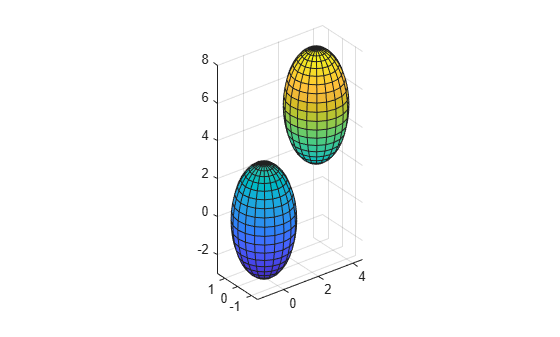Rotate the second ellipsoid by 45 degrees around its $x$-axis. The new coordinates of the translated and rotated ellipsoid are stored in s.Xdata, s.Ydata, and s.Zdata.

direction = [1 0 0];
rotate(s,direction,45)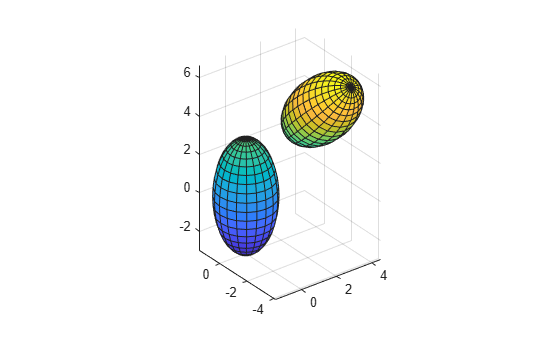Display ellipsoids with center coordinates (0, 0, 0) and semiaxis lengths (2, 1, 1) with different number of faces.

Call the tiledlayout function to create a 2-by-2 tiled chart layout. Call the nexttile function to create the axes. Then, use the ellipsoid function to plot three ellipsoids with different numbers of faces. Plot the ellipsoids in different tiles of the chart by specifying the axes.

tiledlayout(2,2);
ax1 = nexttile;
ellipsoid(ax1,0,0,0,2,1,1)
axis equal
title('20-by-20 faces (Default)')

ax2 = nexttile;
ellipsoid(ax2,0,0,0,2,1,1,50)
axis equal
title('50-by-50 faces')

ax3 = nexttile;
ellipsoid(ax3,0,0,0,2,1,1,80)
axis equal
title('80-by-80 faces')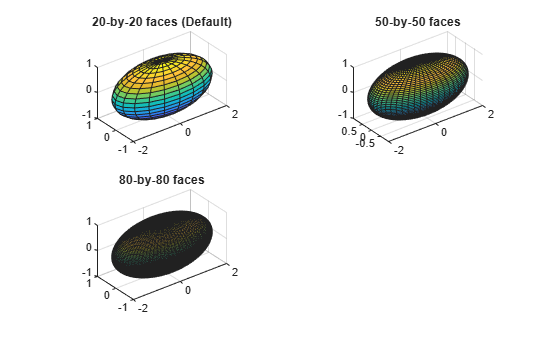Input Arguments

collapse all

Coordinates of ellipsoid center, specified as three comma-separated scalar numbers.

Data Types: single | double

Principal semiaxes along the x-, y-, and z-axes, specified as three comma-separated scalar numbers.

Data Types: single | double

Number of faces, specified as a positive scalar integer.

Target axes, specified as an Axes object. If you do not specify the axes, then ellipsoid plots into the current axes.

Algorithms

ellipsoid generates the data using this equation:

$\frac{{\left(x-xc\right)}^{2}}{x{r}^{2}}+\frac{{\left(y-yc\right)}^{2}}{y{r}^{2}}+\frac{{\left(z-zc\right)}^{2}}{z{r}^{2}}=1.$

ellipsoid(0,0,0,1,1,1) is equivalent to a unit sphere.